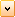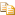WelcomeEvaluate Method (ISurface)

Evaluates the surface, given the u and v parameters of the surface.

# .NET Syntax

Visual Basic (Declaration)
```Function Evaluate( _
ByVal UParam As System.Double, _
ByVal VParam As System.Double, _
ByVal NumUDeriv As System.Integer, _
ByVal NumVDeriv As System.Integer _
) As System.Object```
Visual Basic (Usage)
```Dim instance As ISurface
Dim UParam As System.Double
Dim VParam As System.Double
Dim NumUDeriv As System.Integer
Dim NumVDeriv As System.Integer
Dim value As System.Object

value = instance.Evaluate(UParam, VParam, NumUDeriv, NumVDeriv)```
Csolidworks.interop.sldworks~solidworks.interop.sldworks.isurface~evaluate.html#
```System.object Evaluate(
System.double UParam,
System.double VParam,
System.int NumUDeriv,
System.int NumVDeriv
)```
C++/CLI
```System.Object^ Evaluate(
&   System.double UParam,
&   System.double VParam,
&   System.int NumUDeriv,
&   System.int NumVDeriv
) ```

#### Parameters

UParam

Value of u parameter

VParam

Value of v parameter

NumUDeriv

Number of u derivatives required

NumVDeriv

Number of v derivatives required

#### Return Value

This method returns the evaluated point, normal, and derivatives with respect to u and v up to order NumUDerivs and NumVDerivs, respectively. If NumUDerivs and NumVDerivs are 0, then no derivatives are returned.

For more information on the valid u and v parameters of the surface, see ISurface::Parameterization or ISurface::IParameterization. Also, use IFace2::FaceInSurfaceSense to check to see if the normal of the face is in the same direction or in the opposite direction of the surface normal.

The evaluation returns the following array of (3 *((( NumUDerivs +1) * (NumVDerivs + 1)) + 1 )) doubles:

[ evaluatedPoint, evaluatedDerivatives[xx], evaluatedNormal ]

where:

evaluatedPoint[3= point representing the evaluated X,Y,Z point in meters

evaluatedDerivatives[xx= array of vectors representing the derivatives

where the nth derivative wrt u (i <= NumUDerivs)

and the nth derivative wrt v (j <= NumVDerivs)

would be vector number ( i + (NumUDerivs + 1) * j ) - 1 in the evaluatedDerivatives array.

If NumUDerivs and NumVDerivs are 0, then no derivatives are returned.

evaluatedNormal[3= a vector representing the evaluated normal

The following table describes the number of derivatives that can be requested based on the surface type. If you request more derivatives than allowed, then this method returns all data as zeros. To determine the surface type, see ISurface::Identity.

 U Derivatives
 0 1 2 0 a a b V Derivatives 1 a a c 2 b c d

where:

• a - All surface types

• b - All surface types except blend surfaces

• c - All surface types except blend surfaces and offset surfaces

• d - All surface types except blend surfaces but offset surfaces will only return 0th, 1st, and 2nd derivatives (the uncalculated 3rd and 4th derivatives are returned as 0 vectors).

For example, if you specified 2 derivatives in both u and v, then an array containing 10 vectors is returned. The returned data is in model space coordinates.

[P(u,v)               P(u,v)/du           P(u,v)/dudu]

[P(u,v)/dv           P(u,v)/dudv        P(u,v)/dududv]

[P(u,v)/dvdv        P(u,v)/dudvdv     P(u,v)/dududvdv]

[N(u,v)]

where:

• du the partial derivative in the u direction

• dv the partial derivative in the v direction

• N(u,v) the normal vector

• Each P() represents a model space point or vector depending on the entry in the array

If you request 2 derivatives for u and 1 for v, then an array containing 7 vectors is returned:

[P(u,v)               P(u,v)/du           P(u,v)/dudu]

[P(u,v)/dv           P(u,v)/dudv        P(u,v)/dududv]

[N(u,v)]

If u = 1 and v = 2, then:

[P(u,v)               P(u,v)/du]

[P(u,v)/dv           P(u,v)/dudv]

[P(u,v)/dvdv        P(u,v)/dudvdv]

[N(u,v)]

# ExampleProvide feedback on this topic

* Required

 *Email: Subject: Feedback on Help Topics Page: Evaluate Method (ISurface) *Comment: * I acknowledge I have read and I hereby accept the privacy policy under which my Personal Data will be used by Dassault Systèmes

Print Topic

Select the scope of content to print:

x

We have detected you are using a browser version older than Internet Explorer 7. For optimized display, we suggest upgrading your browser to Internet Explorer 7 or newer.

Never show this message again
x

Web Help Content Version: API Help (English only) 2018 SP05

To disable Web help from within SOLIDWORKS and use local help instead, click Help > Use SOLIDWORKS Web Help.

To report problems encountered with the Web help interface and search, contact your local support representative. To provide feedback on individual help topics, use the “Feedback on this topic” link on the individual topic page.